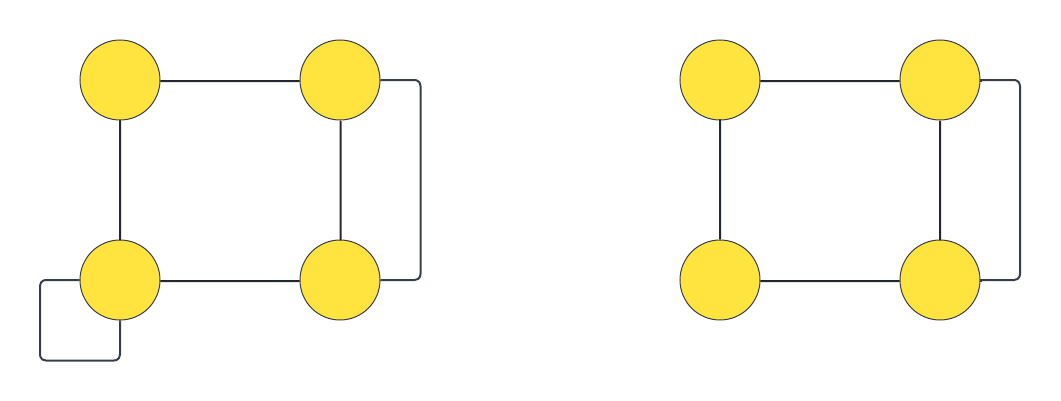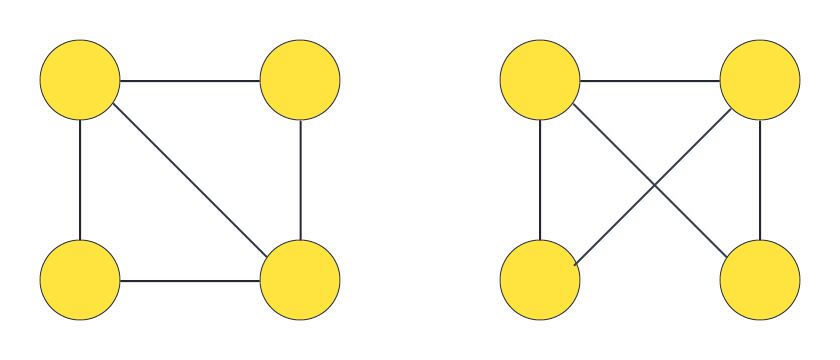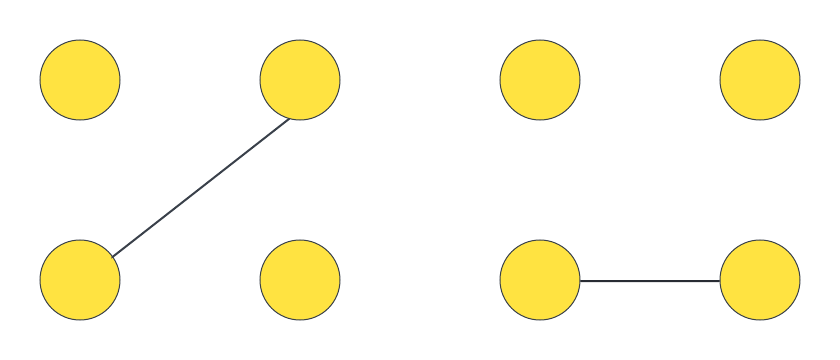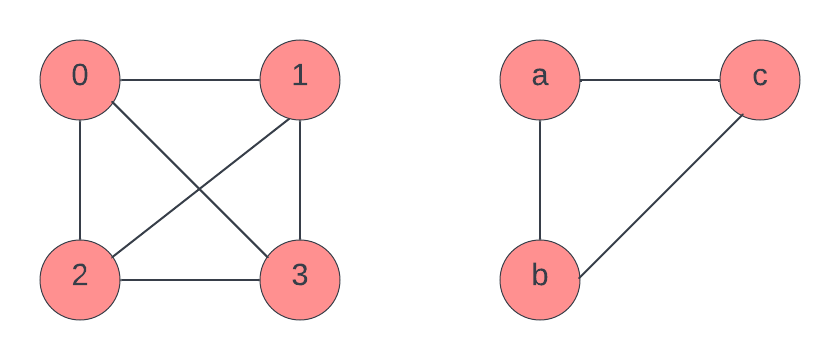×

Search anything:

# Graph and subgraph isomorphism

#### Algorithms Graph AlgorithmsGet this book -> Problems on Array: For Interviews and Competitive Programming

In this article, we will learn about graph and subgraph isomorphism and the algorithms to check for graph and subgraph isomorphism.

1. Graph isomorphism
2. Subgraph isomorphism
3. Subgraph isomorphism algorithms
4. Applications

Graph isomorphism:
Given two simple graphs G and H we can say G and H are isomorphic if there is a one to one correspondence between the vertices and the edges, this means G and H are equivalent in terms of structure.

Conditions for two graphs to be isomorphic:

1. The number of edges in both the graphs should be same.
2. The number of vertices in both the graphs should be the same.
3. The degree sequence which is the sequence of degree of all vertices in ascending order, of both the graphs must be the same.
4. If a cycle of length k can be formed by the vertices in one graph, then a cycle of length k formed by vertices in the second graph should also exist.

The following conditions are not sufficient to call two graphs isomorphic.
Two graphs are isomorphic if and only if their adjacency matrices are same or if their complement graphs are isomorphic.Now we check if the above two graphs are isomorphic.
Number of vertices in graph 1 = 4
Number of vertices in graph 2 = 4

Both graphs have equal number of vertices

Number of edges in graph 1 = 6
Number of edges in graph 2 = 5
Since unequal number of edges, the graphs are not isomorphic.

Checking if the following graphs are isomorphicNumber of vertices in graph 1 = 4
Number of vertices in graph 2 = 4

Number of edges in graph 1 = 5
Number of edges in graph 2 = 5

Degree Sequence of graph 1 = {2, 2, 3, 3}
Degree Sequence of graph 2 = {2, 2, 3, 3}

Graph1 and graph2 both contain 2 cycles of length 3 each.

Since the number of vertices, edges are equal and the degree sequences are also same along with the number and size of the cycles in the graphs, the graphs might be isomorphic since all the above conditions are necessary but not sufficient conditions to call two graphs isomorphic.

Sufficient condition:
Two graphs are isomorphic if and only if their complement graphs are isomorphic.

We can see that the complement graphs are isomorphic.Hence the two graphs- graph1 and graph2 are isomorphic.

• The graph isomorphism problem is the computational problem of determining whether two finite graphs are isomorphic.

• The graph isomorphism problem is neither NP complete, co-NP or P so its in a class of its own called the GI class. The class GI is a set of problems with a polynomial time Turing reduction to the graph isomorphism problem.

• A problem X is called complete for GI, or GI-complete, if it is both GI-hard and a polynomial-time solution to the GI problem would yield a polynomial-time solution to X.

• Whitney graph isomorphism theorem states that two connected graphs are isomorphic if and only if their line graphs are isomorphic.

### Subgraph isomorphism

• The subgraph isomorphism problem also called subgraph matching is a computational problem where two graphs are given as input and it is to determined if one graph contains a subgraph that is isomorphic to the other graph.
• The clique decision problem is reducible to the subgraph isomorphism problem in polynomial time which is NP Complete.
• The subgraph isomorphism problem is NP complete.
• The subgraph isomorphism problem for certain classes of graphs can be solved in polynomial time.

In the following figure, G1 is the first graph and G2 is the second graph.Subgraph of graph G1 is isomorphic to graph G2

f(0) = a
f(1) = c
f(2) = b

The function f is a bijection, hence subgraph of graph G1 is isomorphic to graph G2

### Algorithms

1. Brute force enumeration technique can be used to check for graph isomorphism and subgraph isomorphism.
1. The subgraph isomorphism problem can be represented as a matrix in which each row contains exactly one 1 and each column contains at most one 1.
2. Assume M is the matrix name, now M is of the order m * n where m is the number of nodes in the input graph and n is the number of nodes in the other graph.
3. Now all the possible matrices M are enumerated and isomorphism is checked.
2. Messmer algorithm uses dynamic programming approach and is used to detect subgraph isomorphism.
3. Simulated annealing algorithms, type of evolutionary computation techniques can be used to detect graph isomorphism.
4. Error-correcting A* algorithm can be used to check for subgraph isomorphism. Heuristic techniques can be used to speed up the algorithm or to reduce the time complexity.
Error correcting algorithms use edit operations like insertions, deletions of edges or nodes.
5. Ullmann's algorithm is a modification of the basic enumeration algorithm that uses recursive backtracking, it is used for both graph and subgraph isomorphism.

### Applications

1. The graph isomorphism problem also called graph matching is used in fields like pattern recognition, computer vision, information retrieval, chemistry, networking and so on.
2. The molecular structures can be identified using graph isomorphism where, in the graphs, the nodes represent the atoms and the edges represent the bonds.
3. Subgraph isomorphism algorithms are used in biochemical tools on biochemical data to find similarities between chemical compounds.
4. In bioinformatics, protein structures are compared in biological networks where nodes represent protein and edges represent the interaction between the proteins.
5. In image processing, graph isomorphism algorithms are used to match two different images.
6. Subgraph isomorphism algorithms are used to model social networks and identify the structure through the social network where the node represents a person and the edge represents the relation between the nodes or the people.
7. Computer aided design or computer aided mechanical drawings use subgraph isomorphism algorithms.
8. The tools like electronic design automation (EDA) or electronic computer aided design (ECAD) used to design electronic systems like integrated circuits, printed circuit boards (PCB) use graph isomorphism to check if the particular integrated circuit layout matches the ciruit diagram or schematic originally made.
9. Graph isomorphism algorithms are used in finger print matching.#### Sai Siri Chandana Namala

Graduate student of Computer Science at JNTUH College Of Engineering